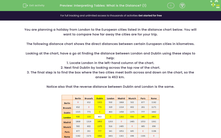### Comprehensive & curriculum aligned

In this worksheet, students will learn how to complete tables that represent quadratic graphs.Key stage:  KS 3

Curriculum topic:   Algebra

Curriculum subtopic:   Use Linear and Quadratic Graphs (One Variable)

Difficulty level:#### Worksheet Overview

When plotting the graph of a quadratic equation, it's useful to draw out and complete a table so that we can find our co-ordinates to plot.

The x values are fixed and the corresponding y values are calculated.

Example:

Complete the following graph table:In the table above the x values range from -3 to +3.

To calculate our values for the y co-ordinates we need to substitute the given values of x into the equation for the quadratic curve.

Likewise, if we are given the y co-ordinates we can substitute these values into the equation and solve.

Method

A = x2 when x = -1

Therefore, A = (-1)2 = 1

B = 1 - 2 = -1

C = 0 - 2 = -2

D = x2 when x = 1 so D = (1)2 = 1

E = 4 - 2 = 2

F = -2 like the rest of that row

G = x2 - 2 when x = 3 so G = (3)2 - 2 = 9 - 2 = 7

Let's consolidate this knowledge by working through some questions together.

### What is EdPlace?

We're your National Curriculum aligned online education content provider helping each child succeed in English, maths and science from year 1 to GCSE. With an EdPlace account you’ll be able to track and measure progress, helping each child achieve their best. We build confidence and attainment by personalising each child’s learning at a level that suits them.

Get started